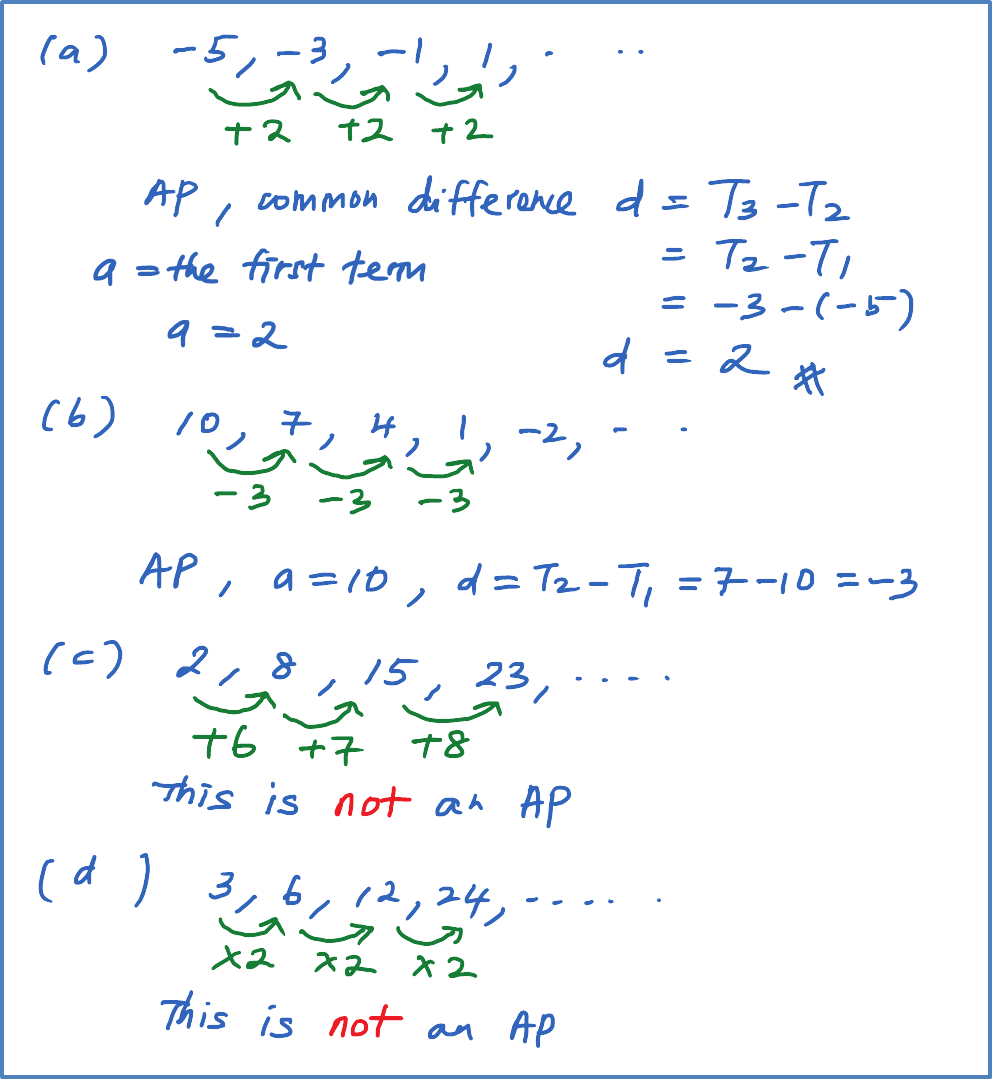\

# 5.2.1 Characteristics Of Arithmetic Progression

Progression

5.2.1 Arithmetic progression
(A) Characteristics of Arithmetic Progression
An arithmetic progression is a progression in which the difference between any term and the immediate term before is a constant.  The constant is called the common difference, d.

 d = Tn – Tn-1   or  d = Tn+1 – Tn

Example 1:

Determine whether the following number sequences is an arithmetic progression (AP) or not.
(a) –5, –3, –1, 1, …
(b) 10, 7, 4, 1, -2, …
(c) 2, 8, 15, 23, …
(d) 3, 6, 12, 24, …

Smart TIPS: For an arithmetic progression, you always plus or minus a fixed number

Solution:(B) The steps to prove whether a given number sequence is an arithmetic progression

Step 1: List down any three consecutive terms. [Example: T1 , T2 , T3 .]
Step 2: Calculate the values of T3  T2 and T2  T1 .
Step 3: If T3  T2 = T2  T1 = d, then the number sequence is an arithmetic progression.
[Try Question 8 and 9 in SPM Practice 1 (Arithmetic Progression)]

Example 2:

Prove whether the following number sequence is an arithmetic progression
(a) 7, 10, 13, …
(b) –20, –15, –9, …

Solution:
(a)
7, 10, 13 ← (Step 1: List down T1 , T2 , T3 )
T3 T2 = 13 – 10 = 3(Step 2: Find T3 T2 and T2 T1)
T2 T1 = 10 – 7 = 3(Step 2: Find T3 T2 and T2 T1)
T3 T2 = T2 T1
Therefore, this is an arithmetic progression.

(b)
–20, –15, –9
T3 T2 = –9 – (–15) = 6
T2 T1 = –15 – (–20) = 5
T3 T2T2 T1
Therefore, this is not an arithmetic progression.